# HSSlive: Plus One & Plus Two Notes & Solutions for Kerala State Board

## AP Board Class 7 Maths Chapter 3 Simple Equations Ex 3.3 Textbook Solutions PDF: Download Andhra Pradesh Board STD 7th Maths Chapter 3 Simple Equations Ex 3.3 Book AnswersAP Board Class 7 Maths Chapter 3 Simple Equations Ex 3.3 Textbook Solutions PDF: Download Andhra Pradesh Board STD 7th Maths Chapter 3 Simple Equations Ex 3.3 Book Answers

## Andhra Pradesh State Board Class 7th Maths Chapter 3 Simple Equations Ex 3.3 Books Solutions

 Board AP Board Materials Textbook Solutions/Guide Format DOC/PDF Class 7th Subject Maths Chapters Maths Chapter 3 Simple Equations Ex 3.3 Provider Hsslive

2. Click on the Andhra Pradesh Board Class 7th Maths Chapter 3 Simple Equations Ex 3.3 Answers.
3. Look for your Andhra Pradesh Board STD 7th Maths Chapter 3 Simple Equations Ex 3.3 Textbooks PDF.
4. Now download or read the Andhra Pradesh Board Class 7th Maths Chapter 3 Simple Equations Ex 3.3 Textbook Solutions for PDF Free.

## AP Board Class 7th Maths Chapter 3 Simple Equations Ex 3.3 Textbooks Solutions with Answer PDF Download

Find below the list of all AP Board Class 7th Maths Chapter 3 Simple Equations Ex 3.3 Textbook Solutions for PDF’s for you to download and prepare for the upcoming exams:

Question 1.
Solve the following equations and check the result.
(i) 5x – 17 = 18
Given 5x – 17 = 18
⇒ 5x – 17 + 17 = 18 + 17 (Add 17 on both sides)
⇒ 5x = 35
⇒ 5𝑥5 = 355 (Divide by 5 on both sides)
⇒ x = 7

Check:
Substitute x = 7 in 5x – 17 = 18
LHS = 5x – 17
= 5(7) – 17
= 35 – 17 = 18 = RHS
Hence verified.

(ii) 29 – 7y = 1
Given 29 – 7y = 1
⇒ 29 – 7y – 29 = 1 – 29 (Subtract 29 on both sides)
⇒ – 7y = -28 .
⇒ 7𝑦7 = 287 (Divide by – 7 on both sides)
⇒ y = 4

Check:
Substitute y = 4 in
29 – 7y = 1
LHS = 29 – 7y
= 29 – 7(4)
= 29 – 28 = 1 = RHS
Hence verified.

(iii) a – 2.3 = 1.5
Given a – 2.3 = 1.5
⇒ a – 2.3 + 2.3 = 1.5 + 2.3 (Add 2.3 ort both sides)
⇒ a = 3.8

Check:
Substitute a = 3.8 in a – 2.3 =1.5
LHS = a – 2.3
= 3.8 – 2.3
= 1.5 = RHS
Hence verified.

(iv) b + 312 = 74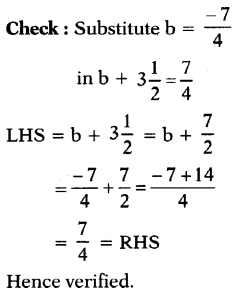(v) 7𝑝10 + 9 = 15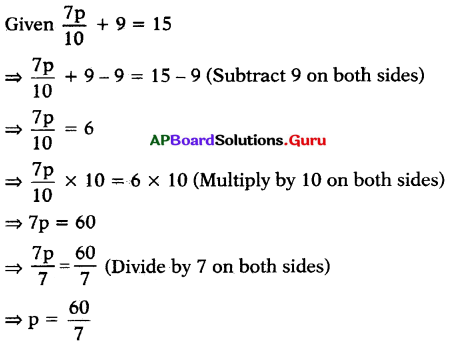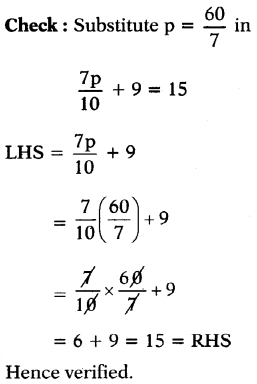(vi) 6(q – 5) = 42.
Given 6(q – 5) = 42
⇒ 6(𝑞5)6 = 426 (Divide by 6 on both sides)
⇒ q – 5 = 7
⇒ q – 5 + 5 = 7 + 5 (Add 5 on both sides)
⇒ q = 12

Check:
Substitute q = 12 in
6(q – 5) = 42
LHS = 6(q – 5)
= 6(12 – 5)
= 6(7) = 42 = RHS
Hence verified.

(vii) – 3(m + 5) + 1 = 13
Given – 3(m + 5)4-1 = 13
⇒ – 3(m + 5)+ 1 – 1 = 13 – 1 (Subtract 1 on both sides)
⇒ – 3(m + 5) = 12
⇒ 3(𝑚+5)3 = 123 (Divide by – 3 on both sides)
⇒ m + 5 = – 4
⇒ m + 5 – 5 = – 4 – 5 (Subtract 5 on both sides)
⇒ m = – 9

Check:
Substitute m = – 9 in – 3(m + 5) + 1 = 13
LHS = – 3(m + 5) + 1
= – 3(- 9 + 5) + 1 = (- 3 × – 4) + 1
= + 12 + 1 = 13 = RHS
Hence verified.

(viii) 𝑛2+𝑛3+𝑛5 = 31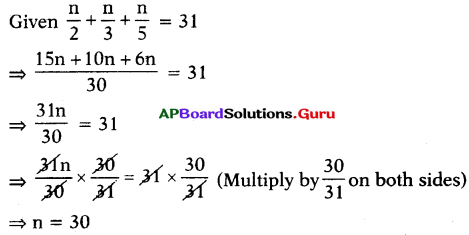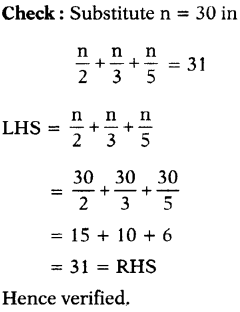Question 2.
Solve the following equations and check the result.

(i) 3(p – 7) – 4 = 5
Given 3(p – 7) – 4 = 5
⇒ 3(p – 7) – 4 + 4 = 5 + 4 (Add 4 on both sides)
⇒ 3(p – 7) = 9
⇒ 3(𝑝7)3 = 93 (Divide by 3 on both sides)
⇒ p – 7 = 3
⇒ p – 7 + 7 = 3 + 7 (Add 7 on both sides)
⇒ p = 10

Check:
Substitute p = 10 in
3(p – 7) – 4 = 5
LHS = 3(p – 7) – 4.
= 3(10 – 7) – 4.
= 3 × 3 – 4
= 9 – 4 = 5 = RHS
Hence verified.

(ii) 5(q – 3) – 3(q – 2) = 0
Given 5(q – 3) – 3(q – 2) = 0
⇒ 5q – 15 – 3q + 6 = 0 (Distributive property)
⇒ 2q – 9 = 0 .
⇒ 2q – 9 + 9 = 0 + 9 (Add 9 on both sides)
⇒ 2q = 9
⇒ 2q2 = 92 (Divide by 2 on both sides)
⇒ q = 92

Check : Substitute q = 92 in
5(q – 3) – 3(q – 2) = 0
LHS = 5(q – 3) – 3(q – 2)
= 5(923) – 3(922)
= 5(962) – 3(942)
= 5 × 32 – 3 × 52
152152 = 0 = RHS
Hence verified.

(iii) 4x – 0.3x – 1.2 = 0.6
Given 0.4x – 0.3x – 1.2 = 0.6
⇒ 0.1 x – 1.2 = 0.6
⇒ 0.1 x – 1.2 + 1.2 = 0.6 + 1.2 (Add 1.2 on both sides)
⇒ 0.1 x = 1.8
⇒ 1x10 = 1810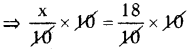(Multiply by 10 on both sides)
⇒ x = 18

Check:
Substitute x = 18 in
0.4x – 0.3x – 1.2 = 0.6
LHS = 0.4x – 0.3x – 1.2
= 0.4(18) – 0.3(18) – 1.2
= 7.2 – 5.4 – 1.2
= 7.2 – 6.6 = 0.6 = RHS
Hence verified.

(iv) 4(3y + 4) = 7.6
Given 4(3y + 4) = 7.6.
⇒ 4(3𝑦+4)4 = 7.64 (Divide by 4 on both sides)
⇒ 3y + 4 = 1.9
⇒3y + 4 – 4= 1.9 – 4 (Subtract 4 on both sides)
⇒ 3y = – 2.1
⇒ 3𝑦3 = – 2.13 (Divide by 3 on both sides)
⇒ y = – 0.7

Check:
Substitute y = – 0.7 in
4(3y + 4) = 7.6
LHS = 4(3y + 4)
= 4[3 (- 0.7) + 4]
= 4[- 2.1 + 4]
= 4 × 1.9 = 7.6 = RHS
Hence verified.

(v) 20 – (2r – 5) = 25
Given 20 – (2r – 5) = 25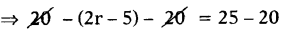(Subtract 20 on both sides)
⇒ – (2r – 5) = 5
⇒ – (2r -5) × – 1 = 5 × – 1
(Multiply by – 1 on both sides)
⇒ 2r – 5 = 5
⇒ 2r – 5 + 5= – 5 + 5 (Add 5 on both sides)
⇒ 2r = 0
⇒ 2r2 = 02 (Divide by 2 on both sides)
⇒ r = 0

Check:
Substitute r = 0 in
20 – (2r – 5) = 25
LHS = 20 – (2r – 5)
= 20 – [2(0) – 5]
= 20 – [0 – 5]
= 20 – (- 5)
= 20 + 5 = 25 = RHS
Hence verified.

(vi) 3(5 – t) – 2(t – 2) = – 1
Given 3(5 – t) – 2(t – 2) = – 1
⇒ 15 – 3t – 2t + 4 .= – 1 (Distributive property)
⇒ 19 – 5t = – 1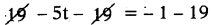(Subtract 19 on both sides)
⇒ – 5t = – 20
⇒ 5𝑡5 = 205 (Divide by – 5 on both sides)
⇒ t = 4

Check:
Substitute t = 4 in
3(5 -1) – 2(t – 2) = – 1
LHS = 3(5 – t) – 2(t – 2)
= 3(5 – 4) – 2(4 – 2)
= 3(1) – 2(2)
= 3 – 4 = – 1 = RHS
Hence verified.

(vii) 3(2k + 1) – 2(k – 5) – 5(5 – 2k) = 16
Given 3(2k + 1) – 2(k T 5) – 5(5 – 2k) = 16
⇒ 6k + 3-2k + 10-25 + 10k = 16 (Distributive property)
⇒ 14k – 12 = 16
⇒ 14k – 12 + 12 – 16 + 12 (Add 12 on both sides)
⇒ 14k = 28
⇒ 14k14 = 2814 (Divide by 14 on both sides)
⇒ k = 2

Check:
Substitute k = 2 in
3(2k + 1) – 2(k – 5) – 5(5 – 2k) = 16
LHS = 3(2k + 1) – 2(k – 5) – 5(5 – 2k)
= 3[2 × 2 + 1] – 2[2 – 5] – 5[5 – 2 × 2]
= 3[4 + 1] – 2(- 3) – 5(5 – 4)
= 15 + 6 – 5 – 16 = RHS
Hence verified.

(viii) 3 m4 – 5m – 34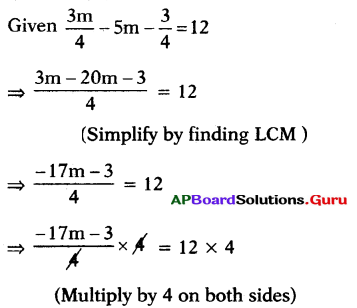⇒ – 17m – 3 = 48
⇒ – 17m – 3 + 3 =48 + 3 (Add 3 on both sides)
⇒ – 17m = 51
⇒ 17 m17 = 5117 (Divide by – 17 on both sides)
⇒ m = – 3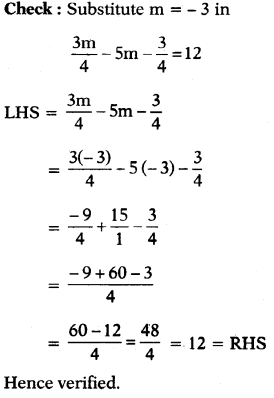(ix) 4𝑛5+𝑛4𝑛2=1110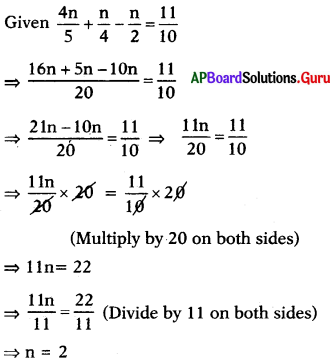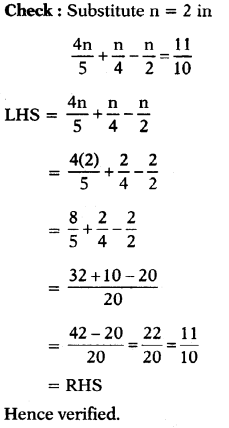(x) 𝑥245+𝑥5+3𝑥10=15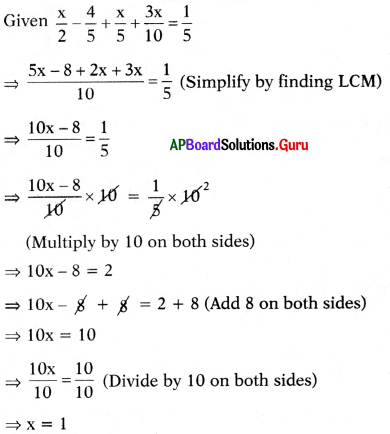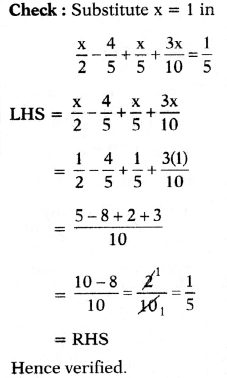Question 3.
Write any three equivalent equations having the solution x = 2.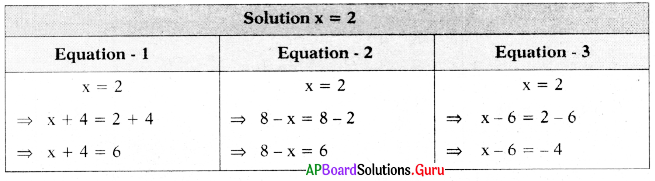Question 4.
Write any three equivalent equations having the solution a = – 5.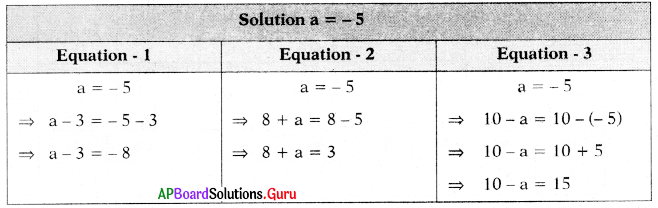## Andhra Pradesh Board Class 7th Maths Chapter 3 Simple Equations Ex 3.3 Textbooks for Exam Preparations

Andhra Pradesh Board Class 7th Maths Chapter 3 Simple Equations Ex 3.3 Textbook Solutions can be of great help in your Andhra Pradesh Board Class 7th Maths Chapter 3 Simple Equations Ex 3.3 exam preparation. The AP Board STD 7th Maths Chapter 3 Simple Equations Ex 3.3 Textbooks study material, used with the English medium textbooks, can help you complete the entire Class 7th Maths Chapter 3 Simple Equations Ex 3.3 Books State Board syllabus with maximum efficiency.

## FAQs Regarding Andhra Pradesh Board Class 7th Maths Chapter 3 Simple Equations Ex 3.3 Textbook Solutions

#### Can we get a Andhra Pradesh State Board Book PDF for all Classes?

Yes you can get Andhra Pradesh Board Text Book PDF for all classes using the links provided in the above article.

## Important Terms

Andhra Pradesh Board Class 7th Maths Chapter 3 Simple Equations Ex 3.3, AP Board Class 7th Maths Chapter 3 Simple Equations Ex 3.3 Textbooks, Andhra Pradesh State Board Class 7th Maths Chapter 3 Simple Equations Ex 3.3, Andhra Pradesh State Board Class 7th Maths Chapter 3 Simple Equations Ex 3.3 Textbook solutions, AP Board Class 7th Maths Chapter 3 Simple Equations Ex 3.3 Textbooks Solutions, Andhra Pradesh Board STD 7th Maths Chapter 3 Simple Equations Ex 3.3, AP Board STD 7th Maths Chapter 3 Simple Equations Ex 3.3 Textbooks, Andhra Pradesh State Board STD 7th Maths Chapter 3 Simple Equations Ex 3.3, Andhra Pradesh State Board STD 7th Maths Chapter 3 Simple Equations Ex 3.3 Textbook solutions, AP Board STD 7th Maths Chapter 3 Simple Equations Ex 3.3 Textbooks Solutions,
Share: